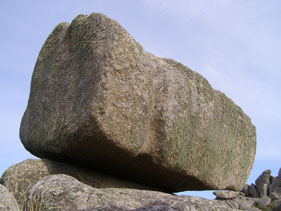µ GCSE | Forces + Weight

10 quick questions - for GCSE and iGCSE

10 minutes maximum! (can you do it in 5?)

Grab a calculator - time for some maths!

Note: Use g = 10 N/kg

1. What are the correct units for force?

• A. joules
• B. newtons
• C. watts
• D. m/s

2. Which of these is the correct formula linking force and acceleration?

• A. force = mass x acceleration
• B. acceleration = mass x force
• C. force = weight x acceleration
• D. acceleration = weight x force

3. What force is needed to make a 2kg firework rocket accelerate at 4 m/s2?

• A. 0.2 N
• B. 2 N
• C. 4 N
• D. 8 N4. A 100g toy car uses an electric motor that produces a force of 3 N. What is the car's acceleration?

• A. 30 m/s2
• B. 300 m/s2
• C. 3 m/s2
• D. 0.3 m/s2

5. When the astronaut Buzz Aldrin first stood on the Moon, he could feel a difference in the pull of gravity. Which statement is correct?

• A. He was weightless.
• B. His mass was now zero.
• C. His mass was the same but his weight was less.
• D. His weight was the same but his mass was less.6. Which of these statements give the correct description and unit for mass and weight?

 Mass (unit) Weight (unit) A Pull of gravity (N) amount of matter (kg) B Pull of gravity (kg) amount of matter (N) C amount of matter (N) Pull of gravity (kg) D amount of matter (kg) Pull of gravity (N)

7. Which of these is the correct formula linking mass and weight?

• A. weight = mass x gravitational field strength ( W = m.g )
• B. weight = mass ÷ gravitational field strength ( W= m/g)
• C. mass = gravitational field strength x weight ( m = g.W )
• D. mass = gravitational field strength ÷ weight ( m = g/W )

Different planets have different strengths of gravity. Rocks on the surface may be easier or harder to lift up.

What are the missing values in the table below?Planet Gravitational Field Strength (N/kg) Mass of rock (kg) weight (N) 8. Mars 4 8 ? 0.5 2 12 32 9. Earth 10 ? 1 100 1000 10000 1000 10. Mercury ? 0.27 0.54 3.7 213 240 888